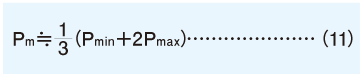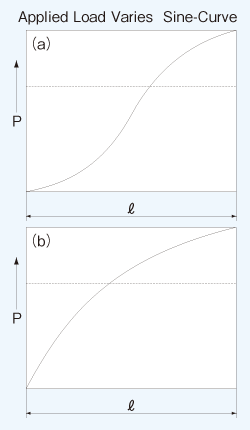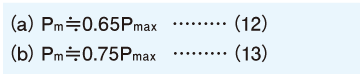HOME > Engineering Info > Life

###### Life of Linear System

When a linear system reciprocates under loading, a continuous stress acts on it, ultimately causing flaking of its race way surface due to material fatigue. The distance a linear system travels before this flaking occurs is defined as the life of the linear system. A linear system can also become inoperable due to sintering, cracking, pitting, or rusting, however, these causes are differentiated from flaking because they are related to installation accuracy, operating environment, and relubrication method.

#### Rated Life

Even when a group of linear systems from the same production lot operated under identical conditions, the life time can differ due to differences in the material fatigue failure characteristics. This fact prevents from determining the exact life time of a single linear system for use. Therefore, the rated life is defined statistically as the distance of 90% of the linear systems travel before causing flaking.

#### Basic Dynamic Load Rating (compliant with ISO14728-1＊2) and Basic Dynamic Torque Rating

＊2: This does not apply to some products.

#### Rated Life Estimation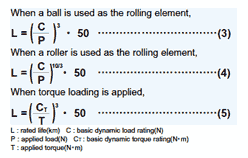The rated life estimation depends on the type of the rolling element. Equations (3) and (4) are used for the ball element and for the roller element, respectively. Equation (5) is used when torque loading is present.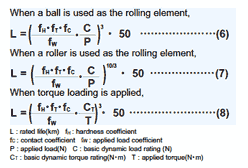In the actual application, numerous variable factors are present such as in guide rail/shaft accuracy, in mounting conditions, in operating conditions, vibration and shock, etc. Therefore, calculating the actual applied load accurately is extremely difficult. In general, the calculation is simplified by using coefficients representing these factors: hardness coefficient (fH), temperature coefficient (fT), contact coefficient (fC), and applied load coefficient (fW). Taking these coefficients into account, Equations (3) to (5) become Equations (6) to (8).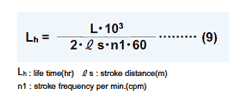When the travel distance per unit time is constant, the rated life can be expressed in terms of time (hour). Equation (9) shows the relationship between stroke length, number of cycles per minute, and the life time.

#### Hardness Coefficient（ｆＨ）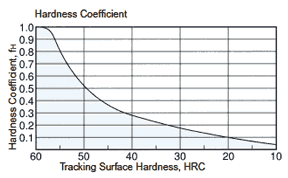In the linear system, the guide rail or shaft works as race way of the rolling elements. Therefore, the hardness of the rail or shaft is an important factor in determining the rated load. The rated load decreases as the hardness decrease below 58HRC. NB products hold appropriate hardness by advanced heat treatment technology. In case of using the rail or shaft of insufficient hardness, please take the hardness coefficient (the right figure) into the life calculation equation.

#### Temperature Coefficient（ｆＴ）In order to give low wear characteristics NB products are hardened by heat treatment. If the temperature of the linear system exceeds 100℃, the hardness is decreased by tempering effect, so as the rated load decreases. the right figure shows the temperature coefficient as hardness changes with temperature.

#### Contact Coefficient（ｆＣ）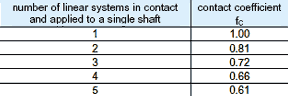When more than one bearing is used in close contact, the contact coefficient should be taken into consideration due to the variation of products and the accuracy of the mounting surface. The right table shows the contact coefficient for life calculation.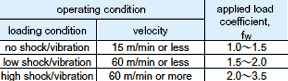When calculating the applied load, the weight of the mass, inertial force, moment resulting from the motion, and the variation with time should be accurately estimated. However, it is very difficult to accurately estimate the applied load due to the existence of numerous variables, including the start/stop conditions of the reciprocating motion and of the shock/vibration. Estimation is simplified by using the values given in the right table.

The tables below show the formulas of applied load calculation for typical applications.
W: applied load (N) P1 - P4: load applied to linear system (N) X,Y: linear system span (mm)
x, y,: distance to applied load or to working center of gravity (mm) g: gravitational acceleration (9.8 x 103mm/s2)
V: velocity (mm/s) t1: acceleration time (sec) t3: deceleration time (sec)

##### Under static conditions or constant velocity motion
 2 horizontal axes applied load calculation formula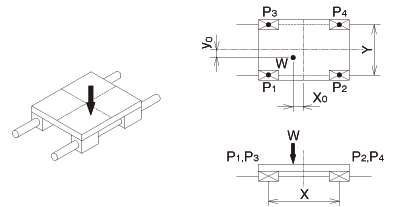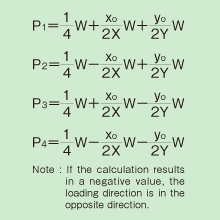2 horizontal axes,over-hang applied load calculation formula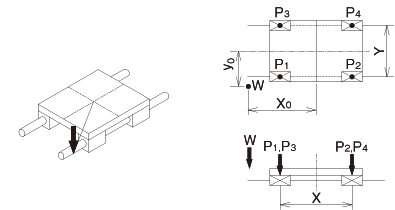2 horizontal axes,moving axes applied load calculation formula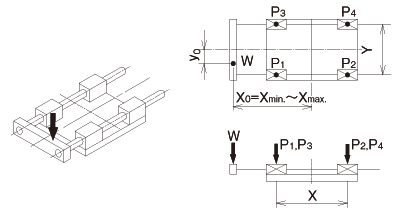2 horizontal, side axes applied load calculation formula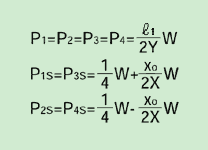2 vertical axes applied load calculation formula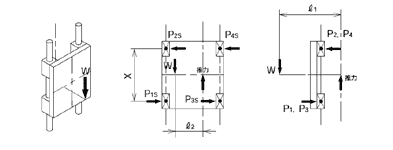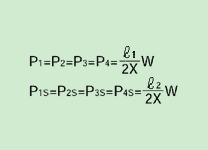##### Under constant acceleration conditions
 2 horizontal axes applied load calculation formula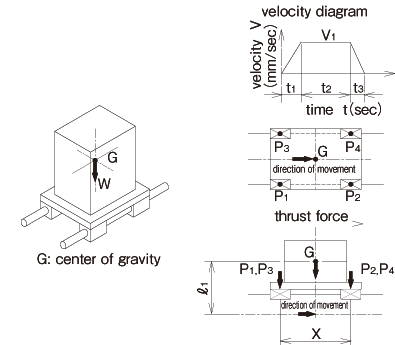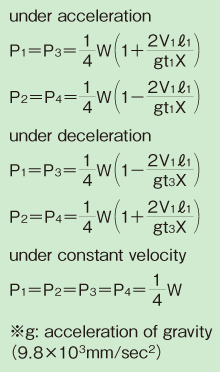##### Equivalent Coefficient

The linear systems are generally used with two axes, each axis with a couple of bearings installed. However, due to a space limitation, there must be an application in which one axis with one or two bearings in close contact installed. In such a case, multiply the applied moment by the equivalent moment coefficient shown in the appended table for applied load calculation. The following is a formula for calculating the equivalent moment load when a moment is applied to the linear system.P: equivalent moment load per bearing（N）
E: equivalent moment coefficient
M: applied moment（N・mm）

The tables below shows the formulas for determining the applied load when moment is applied to the linear system.
W: applied load (N) P: load applied to the linear system (N): distance to applied load or to working center of gravity (mm)

##### 1 axis application
 1 horizontal axis,1 bearing applied load calculation formula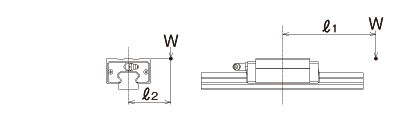1 sideway axis,1 bearing applied load calculation formula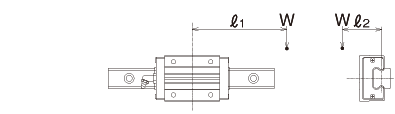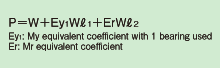1 vertical axis,1 bearing applied load calculation formula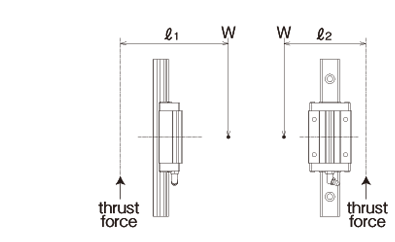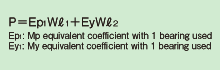##### 2 axes application
 2 horizontal axes,1 bearing each applied load calculation formula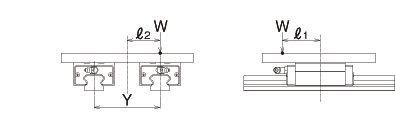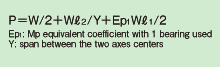2 sideway axes,1 bearing each applied load calculation formula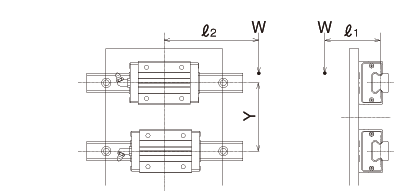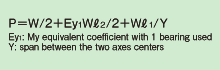2 vertical axes,1 bearing each applied load calculation formula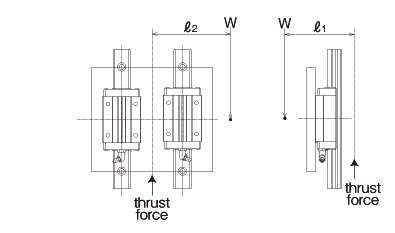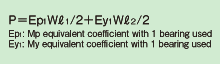The load applied to a linear system generally varies with the travel distance depending on how the system is operated. This includes the start/stop processes of the reciprocating motion and work on the system. The average applied load is used to compute the life corresponding to the actual application conditions.

##### When the load varies in a step manner with the travel distance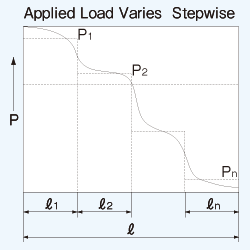1 is the travel distance under load2 is the travel distance under load

・　　　　　　　　　　　　　　　・
・　　　　　　　　　　　　　　　・
・　　　　　　　　　　　　　　　・n is the travel distance under load Pn
The average applied load Pm is obtained by the following equation.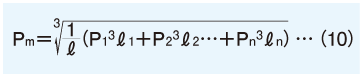: total travel distance (m)

##### When the applied load varies linearly with the travel distanceThe average applied load Pm is a p proximated by the following equation.